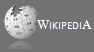Completely Solved C, C++ Programs Assignment.Quick Search Database Videos Tutorials Ebooks Forums FAQ Aboutus Household Industrial Manufacturing Service Shopping Transportation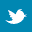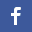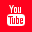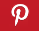### Data structure(Infix,Prefix,Postfix)-PART1

 Filed Under: Moved to New Place

Converting and Evaluating Infix, Postfix and Prefix Expressions in C

 I. INTRODUCTION
Consider a situation where you are writing a programmable calculator. If the user types in 10 + 10, how would you compute a result of the expression? You might have a solution for this simple expression. But what if he types in 3 * 2 / (5 + 45) % 10 ?
What would you do then?
There's no need to think about how you're going to compute the result because no matter what you do, it won't be the best algorithm for calculating expressions. That is because there is a lot of overhead in computing the result for expressions which are expressed in this form; which results in a loss of efficiency.
The expression that you seen above is known as Infix Notation. It is a convention which humans are familiar with and is easier to read. But for a computer, calculating the result from an expression in this form is difficult. Hence the need arises to find another suitable system for representing arithmetic expressions which can be easily processed by computing systems. The Prefix and Postfix Notations make the evaluation procedure really simple.
But since we can't expect the user to type an expression in either prefix or postfix, we must convert the infix expression (specified by the user) into prefix or postfix before processing it.
This means that your program would have to have two separate functions, one to convert the infix expression to post or prefix and the second to compute the result from the converted expression.